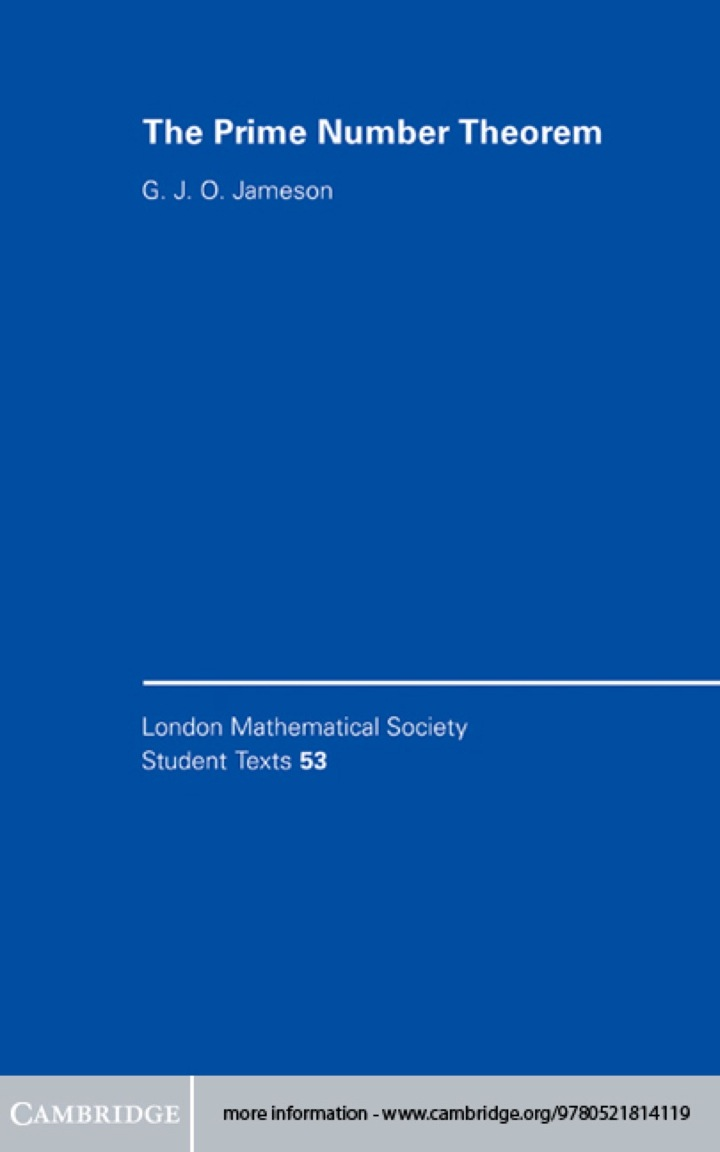# The Prime Number Theorem

## The Prime Number Theorem

By G. J. O. Jameson

### Rent or Buy eTextbook

Expires on Apr 11th, 2022
\$45

Publisher List Price: \$0.00

At first glance the prime numbers appear to be distributed in a very irregular way amongst the integers, but it is possible to produce a simple formula that tells us (in an approximate but well defined sense) how many primes we can expect to find that are less than any integer we might choose. The prime number theorem tells us what this formula is and it is indisputably one of the great classical theorems of mathematics. This textbook gives an introduction to the prime number theorem suitable for advanced undergraduates and beginning graduate students. The author's aim is to show the reader how the tools of analysis can be used in number theory to attack a 'real' problem, and it is based on his own experiences of teaching this material.

Subject: Mathematics & Statistics -> Post-Calculus -> Number Theory1st edition
Publisher: Cambridge University Press 4/17/03
Imprint: Cambridge University Press
Language: English

ISBN 10: 0511075081
ISBN 13: 9780511075087
Print ISBN: 9780521814119

Live Chats